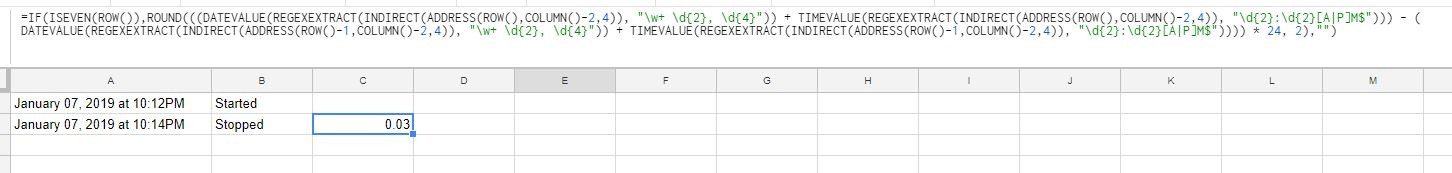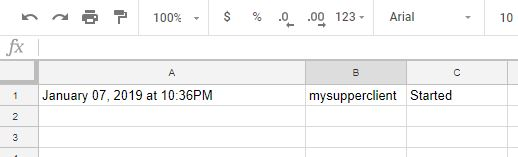January 08, 2019

Working alone at home needs some particular tools. Everyday I have to track spending time on each task I do. Here is my self made tool.

Two years ago, I published an experimentation about a timeseries DB like using Spreadsheet and a Google Script :

Using a DB (relational or not) for this usage is an overkill solution. I need the simpliest tool, reliable and easy to deploy.

The idea is to log every day work and keep an history on what I did for each of my clients. When looking at ifttt, I saw a particular interesting applet : Press a button to track work hours in Google Drive The result is almost what I need :Take a look of how it's genious :``` =IF(ISEVEN(ROW()),ROUND(((DATEVALUE(REGEXEXTRACT(INDIRECT(ADDRESS(ROW(),COLUMN()-2,4)), "\w+ \d{2}, \d{4}")) + TIMEVALUE(REGEXEXTRACT(INDIRECT(ADDRESS(ROW(),COLUMN()-2,4)), "\d{2}:\d{2}[A|P]M\$"))) - ( DATEVALUE(REGEXEXTRACT(INDIRECT(ADDRESS(ROW()-1,COLUMN()-2,4)), "\w+ \d{2}, \d{4}")) + TIMEVALUE(REGEXEXTRACT(INDIRECT(ADDRESS(ROW()-1,COLUMN()-2,4)), "\d{2}:\d{2}[A|P]M\$")))) * 24, 2),"") ```

I found 2 main problems :

• There is no title for the task, only start and stop date/time
• Using my mobile phone or one of my tablets around my space work is not (for me) a good solution.

Take a look at the Google Spreadsheet action available in IFTTT :

I created an improved version with email, I'll send an email with the task name I'm starting as subject (for example).Awesome ! It has all I need ! Sending an email with the task in the subject. Maybe I need another trigger to stop the timer.# Use Google Assistant as a trigger

I have a Google Home Mini. It was a birthday present with a small paper on it : hack it ! Well, hacking with IFTTT is not the greatest hack you can make but I is going to be very usefull.

Create a trigger "Google assitant" and choose "Say a phrase with a text ingredient". Use the same action with a little change on the text field.``` {{CreatedAt}} ||| {{TextField}} ||| =IF(ISODD(ROW()), "Started", "Stopped") ||| =IF(ISEVEN(ROW()),ROUND(((DATEVALUE(REGEXEXTRACT(INDIRECT(ADDRESS(ROW(),COLUMN()-2,4)), "\w+ \d{2}, \d{4}")) + TIMEVALUE(REGEXEXTRACT(INDIRECT(ADDRESS(ROW(),COLUMN()-2,4)), "\d{2}:\d{2}[A|P]M\$"))) - ( DATEVALUE(REGEXEXTRACT(INDIRECT(ADDRESS(ROW()-1,COLUMN()-2,4)), "\w+ \d{2}, \d{4}")) + TIMEVALUE(REGEXEXTRACT(INDIRECT(ADDRESS(ROW()-1,COLUMN()-2,4)), "\d{2}:\d{2}[A|P]M\$")))) * 24, 2),"") ```

For a more natural way of speaking, I made another voice trigger, I have to say "finished !" and it takes the previous text field. Because I do not have the title of my task as a ingredient, I put this formula :

``` =INDIRECT("B" & ROW() - 1) ```

I'll see in a few monthes if this little hack is the right way for me. I spent only two hours on it.